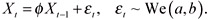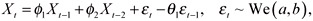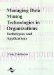# Simulation Studies

 < Day Day Up >

Here we conduct simulation studies to show empirically that the predictive mode forecast has the least root mean square error (RMSE) on average. Two simulation models are considered.

#### Case 1.

In case 1, we generate a first order autoregressive series (AR(1)) of length 400 and parameter values equal to 0.3, 0.5, 0.7 and 0.9, respectively. This simulated time series is driven by the Weibull innovations. That is

 (20)The first 150 observations are discarded to avoid initial transient phenomena. Then we use the series of length 200 to obtain next 10 one-step ahead forecasts with four individual methods. The first method is the forecast produced by the AR(1) model (the true model). The second is the forecast by the naive method. The third is the forecast produced by a moving average model of order five and the final method is the forecast by the exponential smoothing method with smoothing parameter, a = 0.5.

At each forecasting step, we use the four individual forecast values to generate the predictive mode forecast by using the Gibbs sampler scheme. At each step, we generate 20,000 predictive values in the chain. Then the mode of the empirical predictive distribution is obtained by using Eq. (19). The whole process is repeated 1,000 times. Of course, the best performer is the forecast by the AR(1) model since this is the true model which generated the data. The simulation results of 1,000 runs for the AR(1) series with parameter φ = 0.5 are presented in Table 1. Table 1 also includes the results of the three combination forecast methods mentioned in "Individual and Combination Forecasts." Similarresults are obtained for other parameter values.

Table 1: Results for case 1 simulation study

Forecasting Method

Number of Runs

Mean RMSE

S.E.

AR(1) Method

1,000

8.4853

3.18

Predictive Mode

1,000

13.4655

3.37

Naive Method

1,000

15.7872

3.94

MA(5) Method

1,000

17.8885

4.86

Exp. Smoothing Method

1,000

15.1657

4.78

Simple Average Method

1,000

14.9666

3.39

Grange Method (G1)

1,000

14.5945

3.78

Grange Method (G2)

1,000

14.1572

3.96

#### Case 2.

In case 2, we generate time series data by using an ARMA(2,1) model, also of length 400. That is

 (21)with φ1 = 0.5, φ2 = 0.3 and θ1 = 0.3. Again the first 150 observations are also discarded to avoid initial transient phenomena. The simulation runs are exactly the same as in Case 1 except we use data generated by an ARMA(2, 1) model and we have not used the true model to forecast. We used the AR(1) model instead. The simulation results of the 1,000 runs are presented in Table 2. The final results indicate that the mode forecast is the best in terms of the RMSE values among the individual forecast methods as well as the three combination forecast methods. Similar results are obtained for φ1 = 0.3, φ2 = 0.3 and θ1 = 0.2.

Table 2: Results for case 2 simulation study

Forecasting Method

Number of Runs

Mean RMSE

S.E.

Predictive Mode

1,000

14.8222 [a]

3.34

AR(1) Method

1,000

27.2778

4.33

Naive Method

1,000

18.3031

4.93

MA(5) Method

1,000

15.5415

3.61

Exp. Smoothing Method

1,000

15.9643

3.39

Simple Average Method

1,000

19.0292

3.98

Grange Method (G1)

1,000

17.5978

3.68

Grange Method (G2)

1,000

16.9325

3.45

[a]The predictive mode method has the minimum RMSE value.

 < Day Day Up >Managing Data Mining Technologies in Organizations: Techniques and Applications
ISBN: 1591400570
EAN: 2147483647
Year: 2003
Pages: 174